Refer to our Texas Go Math Grade 1 Answer Key Pdf to score good marks in the exams. Test yourself by practicing the problems from Texas Go Math Grade 1 Module 18 Assessment Answer Key.

Vocabulary

Circle the clock that shows time to the half hour. (p.624)
Underline the clock that shows time to the hour. (p.624)

Question 1.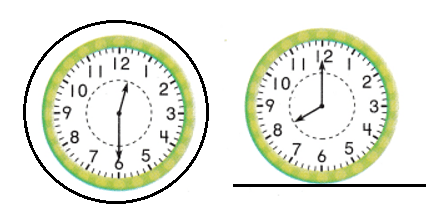Explanation:
First clock shows the time: 12:30 or half past 12:00.
Second clock shows the time: 8:00.

Concept and Skills
Write the time. (TEKS 1.7.E)
Question 2.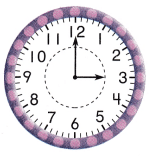Time shown in the clock = 3:00.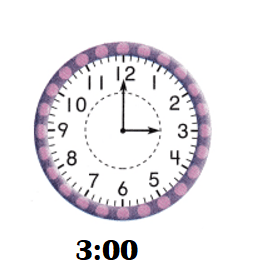Explanation:
Hours hand showing: 3.
Minutes hand showing: 12.

Question 3.Time shown in the clock = 1:30 or half past 1:00.Explanation:
Hours hand showing: 1.
Minutes hand showing: 6.

Write the time. (TEKS 1.7.E)

Question 4.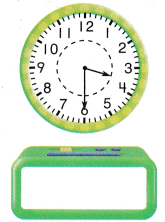Time shown in the clock = 3:30 or half past 3:00.Explanation:
Hours hand showing: 3.
Minutes hand showing: 6.

Question 5.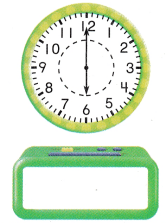Time shown in the clock = 6:00.Explanation:
Hours hand showing: 6.
Minutes hand showing: 12.

Question 6.
What is the time? (TEKS 1.7.E)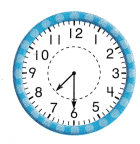(A) 7:00
(B) half past 7:00
(C) 8:00
Time shown in the clock = 7:30 or half past 7:00.
(B) half past 7:00.

Explanation:
Hours hand showing: 7.
Minutes hand showing: 6.Question 7.
What time is it? (TEKS 1.7.E)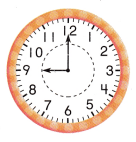(A) 8:00
(B) 9 o’clock
(C) 10 o’clock
Time shown in the clock = 9:00.
(B) 9 o’clock.

Explanation:
Hours hand showing: 9.
Minutes hand showing: 12.Question 8.
Which clock shows 12:30? (TEKS 1.7.E)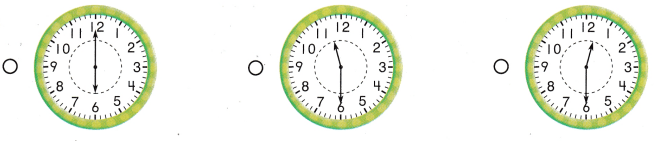Explanation:
Hours hand showing: 6.
Minutes hand showing: 12.
First clock showing the time: 6:00.
Hours hand showing: 11.
Minutes hand showing: 6.
Second clock showing the time: 11:30 or half past 11:00.
Hours hand showing: 12 and 1 between..
Minutes hand showing: 6.
Third clock showing the time: 12:30 or half past 12:00.

Question 9.
Which clock shows 10:00? (TEKS 1.7.E)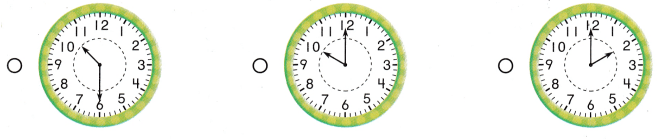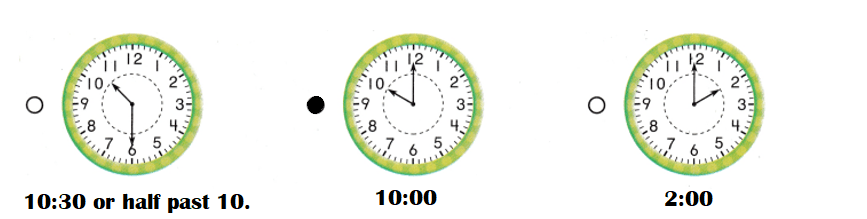Explanation:
Hours hand showing: 10 and 11 between.
Minutes hand showing: 6.
First clock showing the time: 10:30 or half past 10:00.
Hours hand showing: 10.
Minutes hand showing: 12.
Second clock showing the time: 10:00.
Hours hand showing: 2.
Minutes hand showing: 12.
Third clock showing the time: 2:00.

Scroll to Top